Download Presentation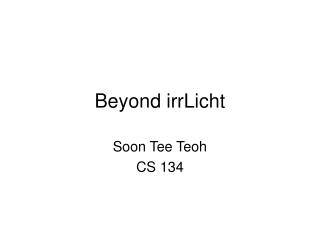Beyond irrLicht

# Beyond irrLicht - PowerPoint PPT Presentation

Beyond irrLicht. Soon Tee Teoh CS 134. Quake 2 MD2 file format. irrLicht examples load the “sydney.md2” file File format is described in http://tfc.duke.free.fr/coding/md2-specs-en.html Each MD2 file contains: Model's geometric data (triangles) Frame-by-frame animationsI am the owner, or an agent authorized to act on behalf of the owner, of the copyrighted work described.
Download Presentation## Beyond irrLicht

An Image/Link below is provided (as is) to download presentation

Download Policy: Content on the Website is provided to you AS IS for your information and personal use and may not be sold / licensed / shared on other websites without getting consent from its author.While downloading, if for some reason you are not able to download a presentation, the publisher may have deleted the file from their server.

- - - - - - - - - - - - - - - - - - - - - - - - - - E N D - - - - - - - - - - - - - - - - - - - - - - - - - -
Presentation Transcript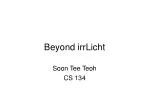### Beyond irrLicht

Soon Tee Teoh

CS 134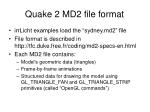Quake 2 MD2 file format
• irrLicht examples load the “sydney.md2” file
• File format is described in http://tfc.duke.free.fr/coding/md2-specs-en.html
• Each MD2 file contains:
• Model's geometric data (triangles)
• Frame-by-frame animations
• Structured data for drawing the model using GL_TRIANGLE_FAN and GL_TRIANGLE_STRIP primitives (called “OpenGL commands”)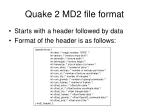Quake 2 MD2 file format
• Starts with a header followed by data
• Format of the header is as follows:

typedef struct {

int ident; /* magic number: "IDP2" */

int version; /* version: must be 8 */

int skinwidth; /* texture width */

int skinheight; /* texture height */

int framesize; /* size in bytes of a frame */

int num_skins; /* number of skins */

int num_vertices; /* number of vertices per frame */

int num_st; /* number of texture coordinates */

int num_tris; /* number of triangles */

int num_glcmds; /* number of opengl commands */

int num_frames; /* number of frames */

int offset_skins; /* offset skin data */

int offset_st; /* offset texture coordinate data */

int offset_tris; /* offset triangle data */

int offset_frames; /* offset frame data */

int offset_glcmds; /* offset OpenGL command data */

int offset_end; /* offset end of file */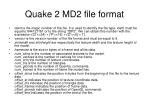Quake 2 MD2 file format
• ident is the magic number of the file. It is used to identify the file type. ident must be equal to 844121161 or to the string “IDP2”. We can obtain this number with the expression (('2'<<24) + ('P'<<16) + ('D'<<8) + 'I').
• version is the version number of the file format and must be equal to 8.
• skinwidth and skinheight are respectively the texture width and the texture height of the model.
• framesize is the size in bytes of a frame and all its data.
• num_skins is the number of associated textures to the model.num_vertices is the number of vertices for one frame.num_st is the number of texture coordinates.num_tris is the number of triangles.num_glcmds is the number of OpenGL commands.num_frames is the number of frame the model has.
• offset_skins indicates the position in bytes from the beginning of the file to the texture data.offset_st indicates the position of texture coordinate data.offset_tris indicates the position of triangle data.offset_frames indicates the position of frame data.offset_glcmds indicates the position of OpenGL commands.offset_end indicates the position of the end of the file.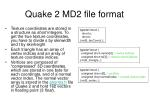Quake 2 MD2 file format
• Texture coordinates are stored in a structure as short integers. To get the true texture coordinates, you have to divide s by skinwidth and t by skinheight
• Each triangle has an array of vertex indices and an array of texture coordinate indices.
• Vertices are composed of “compressed” 3D coordinates, which are stored in one byte for each coordinate, and of a normal vector index. The normal vector array is stored in the anorms.h file of Quake 2 and hold 162 vectors in floating point (3 float).

typedef struct {

short s;

short t;

} md2_texCoord_t;

typedef struct {

unsigned short vertex; // vertex indices

unsigned short st; // texture coord indices

} md2_triangle_t;

typedef struct {

unsigned char v; // position

unsigned char normalIndex; // normal vector index

} md2_vertex_t;Quake 2 MD2 file format
• Frames have specific information for itself and the vertex list of the frame.
• The information is used to uncompress vertices and obtain the real coordinates.
• The vertices of each frame contains the actual coordinates to be drawn.
• To draw each triangle in the frame, get each triangle in the global triangle list to index each vertex in the frame list.

typedef struct {

vec3_t scale; /* scale factor */

vec3_t translate; /* translation vector */

char name; /* frame name */

md2_vertex_t *verts; /* list of frame's vertices */

} md2_frame_t;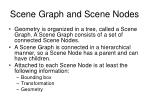Scene Graph and Scene Nodes
• Geometry is organized in a tree, called a Scene Graph. A Scene Graph consists of a set of connected Scene Nodes.
• A Scene Graph is connected in a hierarchical manner, so a Scene Node has a parent and can have children.
• Attached to each Scene Node is at least the following information:
• Bounding box
• Transformation
• Geometry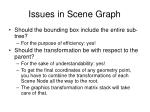Issues in Scene Graph
• Should the bounding box include the entire sub-tree?
• For the purpose of efficiency: yes!
• Should the transformation be with respect to the parent?
• For the sake of understandability: yes!
• To get the final coordinates of any geometry point, you have to combine the transformations of each Scene Node all the way to the root.
• The graphics transformation matrix stack will take care of that.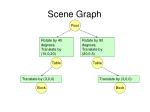Scene Graph

Root

Rotate by 45 degrees, Translate by (10,0,20)

Rotate by 90 degrees, Translate by (20,0,5)

Table

Table

Translate by (3,0,0)

Translate by (3,0,0)

Book

Book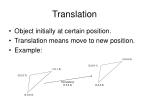Translation
• Object initially at certain position.
• Translation means move to new position.
• Example:

(4.4,4.4)

(3.6,4.1)

(1.0,1.0)

(0.2,0.7)

Translate by

(3.0,0.4)

(3.4,3.4)

(0.0,0.0)Translation
• If the old point is (x,y), and we translate by (tx,ty), the new point is (x+tx,y+ty).
• Works for points, lines, polygons.Rotation
• Have to specify pivot point and rotation angle.

Pivot point: (xr,yr)

Rotation angle: q

q

yr

xr• Let original point (x,y) be:
• x = r cos f, y = r sin f
• New, rotated point:
• x’ = r cos (f + q) = r cos f cos q - r sin f sin q = x cos q – y sin q
• y’ = r sin (f + q) = r cos f sin q + r sin f cos q= x sin q + y cos q

(x’,y’)

(x,y)

q

fScaling
• If the old point is (x,y), and we scale by (sx,sy), the new point is (sxx,syy).
• Works for points, lines, polygons.
• Scale about the origin: the constant pointComposite Transformations
• Translate then rotate is not the same as rotate then translate.
• Translate then scale is not the same as scale then translate.
• Have to specify the order of transformationNot included in irrLicht
• irrLicht is primarily a graphics and collision detection engine.
• For sound, you can use irrKlang
• The irrLicht demo program actually includes irrKlang sound, but you just have to download the irrKlang library and source files separately.
• For physics, refer to some example physics engines on http://irrlicht.sourceforge.net/tutorials.html and how to integrate them with irrLicht.
• Compared to graphics, physics and AI are relatively easy. In following lectures, we’ll cover some algorithms and methods. You can easily convert these to a few lines of code.
• For graphics editor, you can use irrEdit.
• Other editors include (in increasing order of cost and quality): Anim8or, Milkshape, 3D Studio Max and Maya